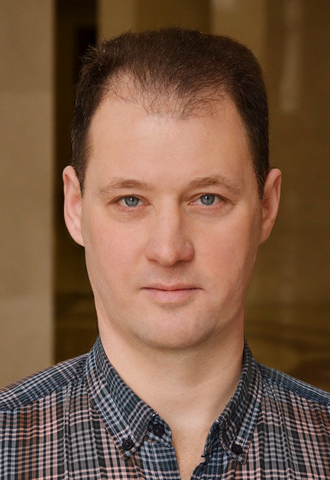# Dmitry Kapilov

### ScheduleCertificate at the International Competition in Pyongyang (Democratic People's Republic of Korea, 2001)

В

Dmitry Kapilov graduated from the Belarusian State Conservatoire in 1997 where he had studied under the PeopleвЂ™s Artist of the USSR A. Savchenko.

He joined the opera company of the Bolshoi Theatre of Belarus in 1997.

From 2003 to 2006, he was a soloist with the Norwegian National Opera and Ballet in Oslo.

### REPERTOIRE

DulcamaraВ В В В В В В В В В В В В В В В В В В В В В В В В В В В В В  L'elisir d'amore

The Old HebrewВ В В В В В В В В В В В В В В В В В В В В  Samson et Dalila

SarastroВ В В В В В В В В В В В В В В В В В В В В В В В В В В В В В В В В В В  Die ZauberflГ¶te

GreminВ В В В В В В В В В В В В В В В В В В В В В В В В В В В В В В В В В В В  Eugene Onegin

King RenГ©, BertrandВ В В В В В В В В В В В В В В  Iolanta

The Prince of Galich, KonchakВ В В В В В В В В В В В В В В  Prince Igor

Figaro, BartoloВ В В В В В В В В В В В В В В В В В В В В В В В  The Marriage of Figaro

Don Basilio, BartoloВ В В В В В В В В В В В В В В  Il barbiere di Siviglia

Escamillo, ZunigaВ В В В В В В В В В В В В В В В В В В  Carmen

ZaccariaВ В В В В В В В В В В В В В В В В В В В В В В В В В В В В В В В В В  Nabucco

The Doctor В В В В В В В В В В В В В В В В В В В В В В В В В В В В В В La traviata

SilvanoВ В В В В В В В В В В В В В В В В В В В В В В В В В В В В В В В В В В  В Un ballo in maschera
FerrandoВ В В В В В В В В В В В В В В В В В В В В В В В В В В В В В В В В В  Il trovatore

The King of EgyptВ В В В В В В В В В В В В В В В В В  Aida
BanquoВ В В В В В В В В В В В В В В В В В В В В В В В В В В В В В В В В В В  Macbeth

Colline, Alcindoro, BenoГ®t В В В В В La bohГЁme

The Bonze, the CommissionerВ В В В В В В В В В В В В В В В  Madama Butterfly

SimoneВ В В В В В В В В В В В В В В В В В В В В В В В В В В В В В В В В В В В  Gianni Schicchi

The Bear В В В В В В В В В В В В В В В В В В В В В В В В В В В В В В В В В The Little Tower Chamber by Ilya Polskiy

The Frost, BermyataВ В В В В В В В В В В В В В В  The Snow Maiden

The MercenaryВ В В В В В В В В В В В В В В В В В В В В В В  The Grey Legend by Dmitry Smolsky

The OwlВ В В В В В В В В В В В В В В В В В В В В В В В В В В В В В В В В  Winnie-the-Pooh by Olga Petrova

BuffВ В В В В В В В В В В В В В В В В В В В В В В В В В В В В В В В В В В В В В В В  Der Schauspieldirektor

The ComposerВ В В В В В В В В В В В В В В В В В В В В В В В  Prima la musica e poi le parole

KhovanskyВ В В В В В В В В В В В В В В В В В В В В В В В В В В В В В  Khovanshchina

DallandВ В В В В В В В В В  В В В В В В В В В В В В В В В В В В В В В В В В В Der fliegende HollГ¤nder
MonteroneВ В В В В В В В В В В В В В В В В В В В В В В В В В В В  В В В Rigoletto

MalyutaВ В В В В В В В В В В В В В В В В В В В В В В В В В В В В В В В В В В  The TsarвЂ™s Bride

The ElephantВ В В В В В В В В В В В В В В В В В В В В В В В В В В  Doctor Ouchithurts by Marina Morozova

SoldiersВ В В В В В В В В В В В В В В В В В В В В В В В В В В В В В В В В В В  Salome

Don PasqualeВ В В В В В В В В В В В В В В В В В В В В В В В В В  Don Pasquale

Lead bass in Requiem by Wolfgang Amadeus Mozart, Requiem by Giuseppe Verdi, Symphony No. 14 by Dmitri Shostakovich

### CONCERT TOUR

Dmitry KapilovвЂ™s international appearances include РњРµlchtal in Guillaume Tell at the Rossini Festival in Germany (conductor Wilhelm Keitel); King RenГ© in Iolanta in Nizhny Novgorod, Russia; Frank in Die Fledermaus in Madrid and Barcelona, Spain; bass part in Johann Sebastian Bach's Magnificat in Bergamo, Oggiono, Milan, Italy.

В

With the company, he has toured Germany, Austria, Spain, Cyprus, the Netherlands, Estonia, and Belgium. В

В

### Partners